Perlin Noise: Pseudorandom functions for terrain generation

The creation of virtual environments requires the modeling of large amounts of training data for different scenarios recreate surfaces. The fractal nature of our world allows the use of various mathematical functions for the automation of these tasks. Trees, land, clouds, fire etc.. are constructed using many different techniques based on the self-similarity, families based complex fractal. The functions that generate the so-called "perlin noise" apply in these cases.We will analyze the concept applied in one dimension, its generalization to two dimensions (land) is immediate, and provides no initial didactic interest.

Noise Functions: “Noise functions (NF)”

A NF is essentially a pseudo random number generator has the property of generating the same number to an input value or "seed" determined.
Any function, such as y = cos(x) always returns the same value of "y" for a particular value of "x".If we use this function to generate a geometric model, we re-obtain the same model later using identical variables. However, the function can not be used in the representation of a profile of dunes or mountains because it has a periodic repetition, a pattern, naturalness which subtracts. Desert dunes resemble each other but are not identical.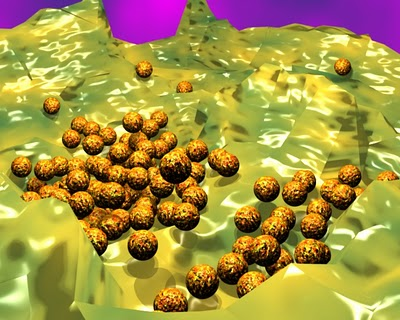A repetitive pattern remains a credible scenario. A pseudorandom pattern can create a profile where you do not identify repetitions.A random function is not controllable do not you can restore or regain previous values, since each time return different values.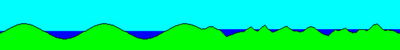A "perlin function" to mix the advantage of the random (noise) with the control of a conventional function. Further intermediate values ​​can be obtained between two values ​​given, providing continuity therefore these functions. If we increase the scale of representation need to get these points in between to keep the resolution of the images generated. If we make a representation at different levels of detail LOD techniques, These aspects are among the most important.

Finally it is necessary to smooth the curve obtained to avoid "peaks" and "valleys" generally very pronounced and generally unwanted unrealistic.
To obtain images at different scales is necessary to maintain the resolution of the same adding new points. The function should allow this operation to ensure a reasonable quality representation.Each function "perlin noise" generated by different data that can adapt better or worse as the desired model creation. This is a field of research currently deep exploration.
In  proposes the use of a number generator psudoaleatorio, based on the use of primes, that returns a number in the range [-1,1] for each integer value that is supplied.

 function Noise (32-bit integer: x) x = (x<<13) ^ x A=15731 B=789221 C=1376312589 return ( 1.0 – ( (x * (x * x * A B) +C) &7fffffff) / 1073741824.0)end Noise function Function "Noise"

The first three numbers are prime. You can experiment with different values ​​to generate new curves. The use of the three variables (A, B to C) was made by clarity of expression.

The code has two main lines.

First "shake" the input value in a controlled manner with the displacement of its bits with

x = (x<<13) ^ x

To generate the number in the above range [-1,1] use the value of its upper end (1) and subtract one value from [0,2].

Obtaining a subtraction value is made by determining a new intermediate value by the use of primes mentioned.

x * ( x * x * A + B) + C

The number generated must be positive, by what logic you mask you put your first bit to zero (positive)

&7fffffff

If we divide this last result would obtain a maximum integer value between zero and one, for it is divided by MaxEntero / 2 = 230

Octaves

To compose the final function adds the "Noise" with other "similar" bending whose frequencies are correspondingly.

The frequency is the inverse of the wavelength F = 1 / L, given notion of periodicity of the waveWhile the amplitude gives us the size (our wave height)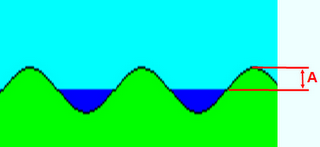Each of these functions previous frequency doubling, similarly to the equal spacing between two consecutive notes on a piano, called "octave".A function and its first octave can compose a new function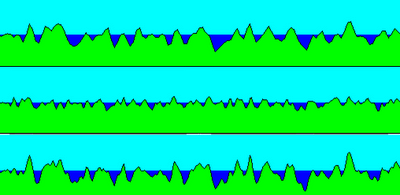A pseudo-random function and its octave added

A pseudo-random function and summed octaves
You can add the harmonics of the function itself or make a mix of similar functions, adding more diversity in the creation of many octaves cornering.The incorporate progressive scale add detail. A greater number of octaves higher complexity in the curve obtained, allowing to simulate steep cliffs.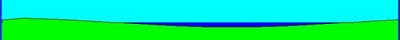12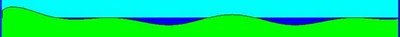3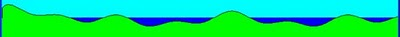4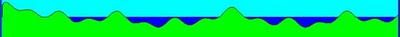5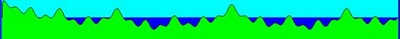6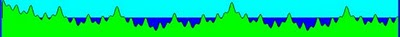7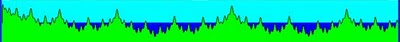89

The persistence factor indicates the incidence or weight of harmonics, adding a control point on the curve smoothness.

Persistence added high values ​​greater change along the curve.

By reducing the value of persistence, the influence of octaves not generate sharp forms.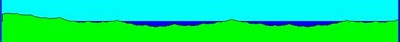For a given frequency is a single amplitude for each value of persistence, we call “Amplitude of each frequency”

Mandelbrot define el ruido, “Noise”, as “High frequency low persistence”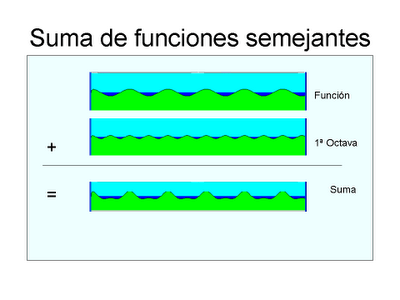The calculation process is performed in several stages.

First we determine the generating function of noise, As explained: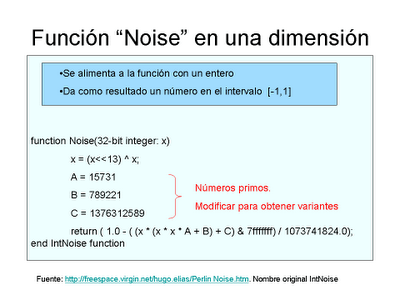Intermediate values ​​between two points can be obtained by linear interpolation, quadratic, cubic etc..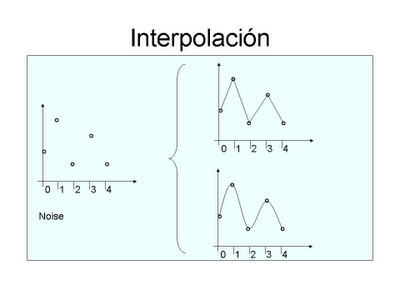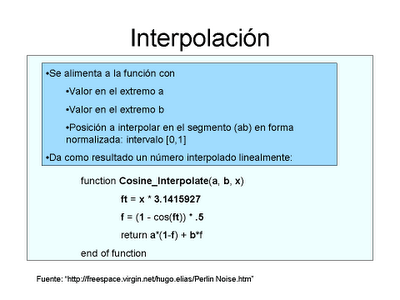Then, is “softens” after the interpolation function: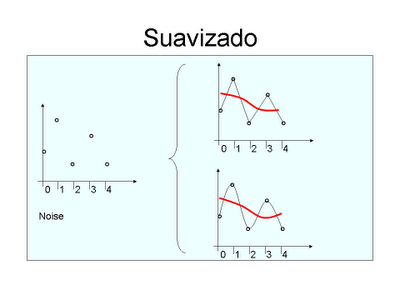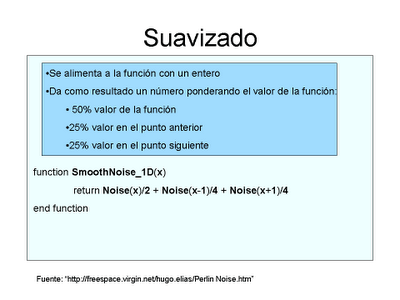And integrating the set, coupling the summing function harmonic components:Page perlin noise explanatory pseudocode. This page is the main reference implementation presented in this work. "http://freespace.virgin.net/hugo.elias/Perlin Noise.htm